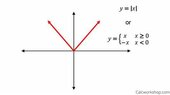# Use Graph To Investigate Limit

Summary:: Use Graph To Investigate Limit

Use the graph to investigate the limit of f(x)
as x tends to 0.

Let me see.

I got to use the graph to investigate the limit of f(x) as x tends to 0 from the left and right.

Let y = f(x).

The given function can also be expressed as f(x) = | x |.

The limit of (-x) as x-->0 from the left is positive infinity.

The limit of (x) as x-->0 from the right is positive infinity.

The limit of f(x) as x-->0 from the left and right at the same time is positive infinity.

Can I say the limit of f(x) is positive infinity?

#### Attachments

•th.jpeg-5.jpg
5.8 KB · Views: 51
Last edited by a moderator:

Gold Member
2022 Award
The limit of (-x) as x-->0 from the left is positive infinity.

The limit of (x) as x-->0 from the right is positive infinity.

The limit of f(x) as x-->0 from the left and right at the same time is positive infinity.

Can I say the limit of f(x) is positive infinity?
Only if you think for some unfathomable reason that zero and positive infinity are the same thing.

You CLEARLY show a graph in which the y value goes to zero as the x value approaches zero. How can you think that this is positive infinity?

Mentor
You CLEARLY show a graph in which the y value goes to zero as the x value approaches zero. How can you think that this is positive infinity?
+1

Only if you think for some unfathomable reason that zero and positive infinity are the same thing.

You CLEARLY show a graph in which the y value goes to zero as the x value approaches zero. How can you think that this is positive infinity?

I was thinking about the V shape formed by the graph of y = | x |. In quadrants 1 and 2, the graph starts at (0, 0) and shoots upward through quadrants 1 and 2 into eternity. However, thinking about it, as I walk toward zero on the x-axis from the left and right, the graph does reach a height of zero. The limit is 0.

+1

Mentor
+1 means I agree with what phinds wrote.
I was thinking about the V shape formed by the graph of y = | x |. In quadrants 1 and 2, the graph starts at (0, 0) and shoots upward through quadrants 1 and 2 into eternity. However, thinking about it, as I walk toward zero on the x-axis from the left and right, the graph does reach a height of zero. The limit is 0.
Since the problem was to find the limit as x approaches zero, it's immaterial what the graph does for large x or for very negative x.

+1 means I agree with what phinds wrote.
Since the problem was to find the limit as x approaches zero, it's immaterial what the graph does for large x or for very negative x.
What about if the graph is y = -| x | as x tends to 0? You said: "...it's immaterial what the graph does for large x or for very negative x." Can you provide an example using another function?

By the way, are you the same MarkFL from Florida? He is very popular in other math forums.

Gold Member
2022 Award
What about if the graph is y = -| x | as x tends to 0? You said: "...it's immaterial what the graph does for large x or for very negative x." Can you provide an example using another function?
Do you have some belief that -0 is different from +0 ?

By the way, are you the same MarkFL from Florida? He is very popular in other math forums.
click on his avatar to see that he is from Washington State

Mentor
What about if the graph is y = -| x | as x tends to 0?
Same limit value.
$$\lim_{x \to 0} |x| = \lim_{x \to 0} -|x| = \lim_{x \to 0} x^2 = \lim_{x \to 0} x^3 = 0$$
You said: "...it's immaterial what the graph does for large x or for very negative x." Can you provide an example using another function?
If you're investigating the limit of some function as x approaches zero, why would you want to check on what happens if x is very large or very negative? That's what I meant by "it's immaterial".

•nycmathguy
Same limit value.
$$\lim_{x \to 0} |x| = \lim_{x \to 0} -|x| = \lim_{x \to 0} x^2 = \lim_{x \to 0} x^3 = 0$$
If you're investigating the limit of some function as x approaches zero, why would you want to check on what happens if x is very large or very negative? That's what I meant by "it's immaterial".

I get it now thanks to you. This is a great site.

Do you have some belief that -0 is different from +0 ?

click on his avatar to see that he is from Washington State

No. Obviously, -0 = +0 = no value. Yes, you are right. He is not MarkFL. I do miss MarkFL. What an incredible mathematician he truly is. Member jonah ruined my friendship with MarkFL. Sorry but I needed to bring this into light.